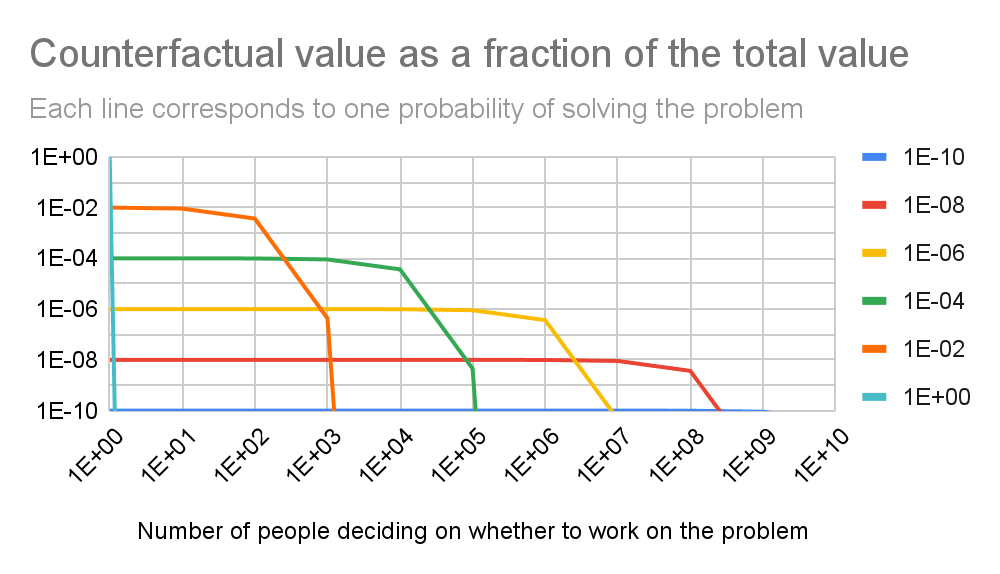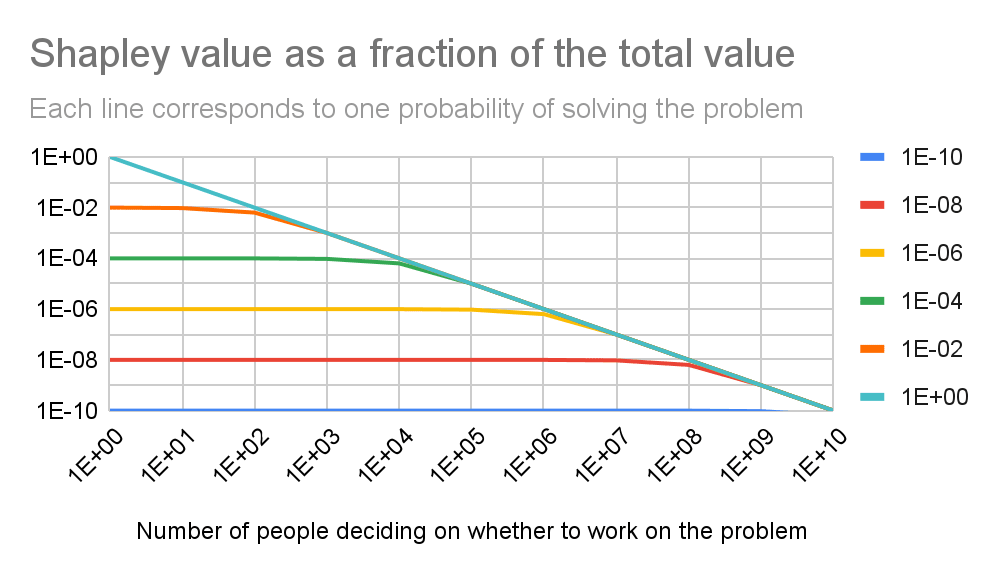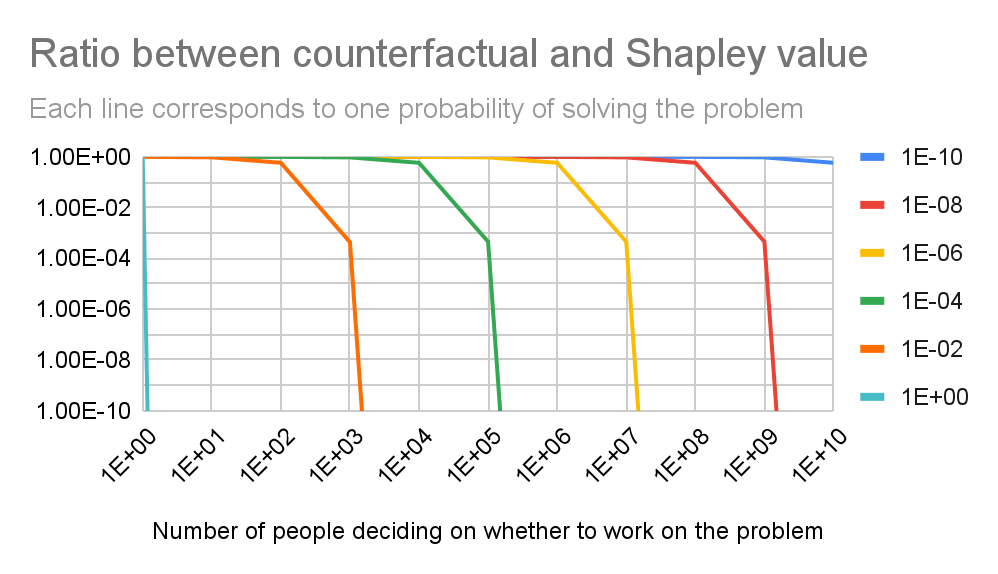# Summary

• I have looked into Shapley and counterfactual value for a number of people working on a problem who each have the same probability of solving it, thus achieving a certain total value.
• The Shapley and counterfactual value of each person is such that diminishing returns only kick in when that probability is high relative to neglectedness, in which case Shapley value is directly proportional to neglectedness.
• Under these conditions, counterfactual value is way smaller than Shapley value, so relying on counterfactuals will tend to underestimate cost-effectiveness when there are more people deciding on whether to work on a problem, like with the effective altruism community.
• Nonetheless, in a situation where a community is coordinating to maximise impact, it might as well define its actions together as a single agent. In this case, Shapley and counterfactual value would be the same. In practice, I guess coordination along those lines will not always be possible, so Shapley values may still be useful.

# Introduction

Shapley values are an extension of counterfactuals, and are uniquely determined by the following properties:

• Property 1: Sum of the values adds up to the total value (Efficiency)
• Property 2: Equal agents have equal value (Symmetry)
• Property 3: Order indifference: it doesn't matter which order you go in (Linearity). Or, in other words, if there are two steps, Value(Step1 + Step2) = Value(Step1) + Value(Step2).

Counterfactuals do not have property 1, and create tricky dynamics around 2 and 3, so Shapley values are arguably better.

In this post, I study a simple model to see how Shapley value relates to the importance, tractability and neglectedness (ITN) framework.

# Methods

For N people who are deciding on whether to work on a problem, and each have a probability p of solving it, which I call easiness, thus achieving a total value V (as I commented here):

• The actual contribution of a coalition with size n is:
• The marginal contribution to a coalition with size n is:
• The counterfactual value of each person is:
• The marginal contribution only depends on the size of the coalition, not on its specific members, and therefore the Shapley value of each person is:

This formula corresponds to the ratio between the total expected value and number of people, given that everyone is in the same conditions. Mapping the Shapley value to the ITN framework:

• The total value would be the importance/scale of the problem.
• The probability of at least one person achieving the total value (denominator of the 1st factor of the formula just above) would be the tractability/solvability.
• The reciprocal of the number of people would be the neglectedness/uncrowdedness.

To facilitate the determination of p and V, the problem could be defined as a subproblem of a cause area, and the value of solving it expressed as a fraction of the importance of that area.

I calculated in this Sheet the counterfactual and Shapley value as a fraction of the total value, and ratio between them for a number of people ranging from 1 to 10^10 (similar to the global population of 8 billion), and probability of achieving the total value from 10^-10 to 1.

# Results# Discussion

The figures show the counterfactual and Shapley value vary as a function of the ratio between easiness and neglectedness (R = p/(1/N)):

• The counterfactual value as a fraction of the total value is:
• 99.0 % of p for R = 0.01.
• 90.5 % of p for R = 0.1.
• 36.8 % of p for R = 1.
• 4.54*10^-5 of p for R = 10.
• Negligible for R >= 100.
• The Shapley value as a fraction of the total value is:
• 100 % of p for R <= 0.01.
• 95.2 % of p for R = 0.1.
• 63.2 % of p for R = 1.
• 10.0 % of p for R = 10.
• Approximately 1/N for R >= 10.
• The ratio between counterfactual and Shapley value is:
• 99.5 % for R = 0.01.
• 95.1 % for R = 0.1.
• 58.2 % for R = 1.
• 0.0454 % for R = 10.
• Negligible for R >= 100.

This illustrates diminishing returns only kick in when easiness is high relative to neglectedness. When easiness is low relative to neglectedness, the Shapley and counterfactual value are essentially equal to the naive expected value (p V) regardless of the specific neglectedness. When easiness is high relative to neglectedness, the Shapley value is directly proportional to neglectedness (SV = V/N), and counterfactual value quickly approaches 0.

Counterfactual value only matches Shapley value if easiness is low relative to neglectedness. When the opposite is true, counterfactual value is way smaller than Shapley value. Consequently, relying on counterfactuals will tend to underestimate (Shapley) cost-effectiveness when there are more people deciding on whether to work on a problem, like with the effective altruism community.

I wonder about the extent to which easiness and neglectedness are independent. If easiness is often high relative to neglectedness (e.g. R >= 10), assuming diminishing returns for all levels of neglectedness may be reasonable. However, in this case, counterfactual value would be much smaller than Shapley value, so using this would be preferable.

In any case, the problem to which my importance, easiness and neglectedness framework is applied should be defined such that it makes sense to model the contribution of each person as a probability of solving it. It may be worth thinking in these terms sometimes, but contributions to solving problems are not binary. Nevertheless, I suppose one can get around this by interpreting the probability of solving the problem as the fraction of it that would be solved in expectation.

Overall, I am still confused about what would be good use cases for Shapley value (if any). Counterfactual value can certainly be misleading, as shown in my 3rd figure, and even better in Nuño’s illustrative examples. Nonetheless, as tobycrisford commented, in a situation where a community is coordinating to maximise impact, it might as well define its actions together as a single agent. In this case (N = 1), Shapley and counterfactual value would be the same (in my models, p V). In practice, I guess coordination along those lines will not always be possible, so Shapley values may still be useful, possibly depending on how easy it is to move towards single-agent coordination relative to building estimation infrastructure.

# Acknowledgements

Thanks to Nuño Sempere for feedback on the draft.

1. ^

Note “tractability” = 1 - (1 - “easiness”)^N.